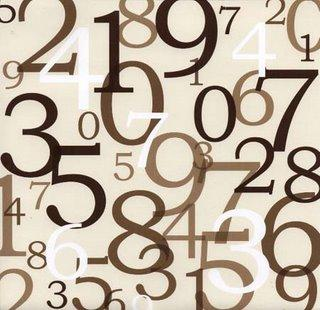# Code To Generate Random Numbers In Java

Generating random number is an essential tool to do some random functions or actions in a program using any programming language. Today in this post i will tell you the easiest way to generate random numbers in JAVA programming language. In Java generating random numbers is such a easier task, all we need to do is import util.Random and to place two little lines of codes which will generate numbers randomly.

What to do?
First of all import util library's Random function. use following code:

`import java.util.Random;`

Then place the following code to get random number.

```Random rand = new Random();
int prob = (int) (100 * rand.nextDouble());```

How it works?
The above code will generate random numbers in the range 0 to 99.
If you want to get numbers in range 0 to 9 :- modify the above code in following way:

```Random rand = new Random();
int prob = (int) (10*rand.nextDouble());```
Even more...
From the above code we can easily generate random numbers, which helps us to do some random functions in our java program and make it more user friendly and professional. Now if your program needs random numbers but continuously repeating of the same number fails your program efficiency! than you must switch to generate random numbers without repeating. I already created a method in java which generates random number without repeating the same number again. Check that code too(below link) :
> Generate Random Numbers Without Repeating